Neural NetworksNeural Networks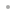FeaturesExamplesTable of ContentsQ&ABuy OnlineQ: How can I see the parameters of a neural network?

The parameters, or synaptic weights as they are also called, are always stored in the first element of a network, as described in 3.2.3: Network Format. The meaning of the individual parameters is better explained in 13.1: Change the Parameter Values of an Existing Network. Most neural networks can be evaluated on a symbolic input vector; then the meaning of the parameters can be illustrated as in the example below.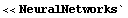Generate a network for demonstration purposes.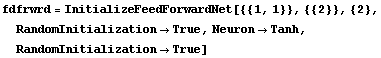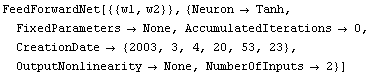The numerical values of the parameters are all placed in the first element of the network.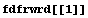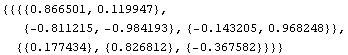You can extract the first element and perform whatever process you want with Mathematica.

An easy way to identify the meaning of the individual parameter is obtained by applying the network to a symbolic input.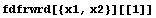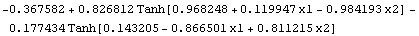If you need to implement the trained network in, for example, a C program, you might find the following command useful.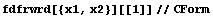-0.36758202492667336 + 0.8268122850621071*
Tanh(0.9682476245672822 + 0.11994745409360652*x1 -
0.9841928909337702*x2) -
0.17743436966754977*Tanh(0.1432051850856072 -
0.866500737817343*x1 + 0.8112145157992191*x2)

Q: Can I use several data sets simultaneously to train a neural network?

For most types of neural networks you concatenate the data sets into one set before the training. This is shown in the first example below. For dynamic neural networks, neural ARX and neural AR, it is slightly more complicated because the individual data items are correlated. How you manage in this case is shown in the second example.

##### Nondynamic Neural Networks

The general data format is described in 3.2.1: Data Format.Generate a network for demonstration purposes.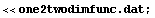In this data file, the input and output matrices are assigned to the variable names x and y respectively. Once the data set has been loaded, you can query the data using Mathematica commands. To better understand the data format and variable name assignment, you may also want to open the data file itself.

Load a data file containing input data x and output data y.Show the contents of the input and output matrices.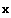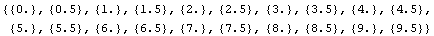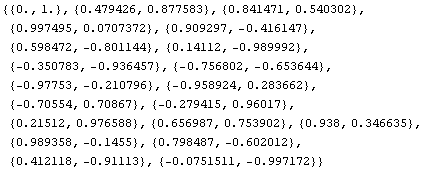Check the number of data items and the number of inputs and outputs for each.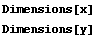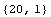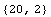There are 20 data samples; the input has one dimension, and the output has two. Introduce a second data set.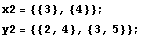The dimensionality of the input and output must be the same in the two data sets.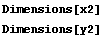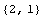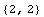A new, joined data set can now be constructed by concatenating the two input data sets, and similarly with the output data sets.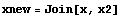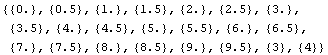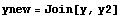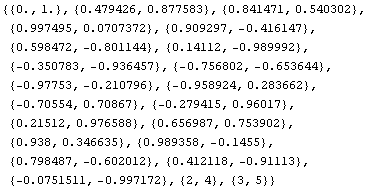Now you can start training your network with the combined data set.

##### Dynamic Neural Networks

Data from dynamic systems are correlated, and if two data sets are concatenated in the way described in the previous example, then a transient is introduced in the data. This transient might be small and unimportant. There is, however, a way to avoid the transient totally. What you have to do is to build the regressor, described in 2.6: Dynamic Neural Networks, for the two data sets and then concatenate the result.

<<NeuralNetworks`

<<Statistics`ContinuousDistributions`

<<Graphics`MultipleListPlot`

For demonstration purposes, generate a first data set.

Ndata = 30;
u1 = RandomArray[NormalDistribution[0, 3], {Ndata, 2}];
x = FoldList[Function[{xs, uin}, {(uin[] + uin[] +
0.6 * xs[] + 0.8 * xs[])/(1 + xs[]^2), 0.7 * xs[] +
uin[], xs[]}], {0, 0, 5}, Drop[u1, -1]];
y1 = x[[All, {1, 2}]];

Check dimensions of input and output, and the number of data for the first data set.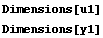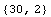Generate a second data set.

Ndata = 20;
u2 = RandomArray[NormalDistribution[0, 3], {Ndata, 2}];
x = FoldList[Function[{xs, uin}, {(uin[] + uin[] +
0.6 * xs[] + 0.8 * xs[])/(1 + xs[]^2), 0.7 * xs[] + uin[], xs[]}], {0, 0, 5}, Drop[u2, -1]];
y2 = x[[All, {1, 2}]];

Check dimensions of input and output, and the number of data for the second data set.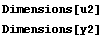Choose the indices indicating the regressor, as described in 2.6: Dynamic Neural Networks.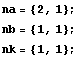With one data set you can train a neural ARX model in the following way.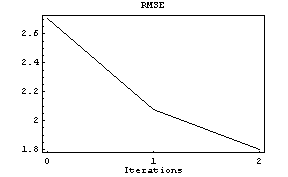With two data sets you have to do the following.

Generate the regressors and modified outputs of the two data sets.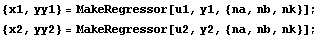Concatenate regressors and the modified outputs in the same way as in the previous example.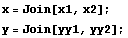Initialize a neural ARX model of your choice (by indicating nil training iterations in NeuralARXFit).Extract the feedforward or the RBF network in the first position of the initialized neural ARX model (as described in 8.1.1: Initializing and Training Dynamic Neural Networks).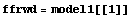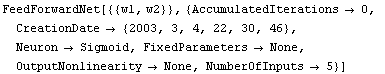Train the feedforward or the RBF network.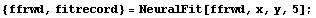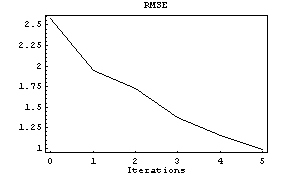Put back the trained network at the first position of the neural ARX model.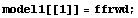You have now obtained a neural ARX model trained on both data sets. The neural ARX model can be used, for example, to simulate the behavior of one of the data sets.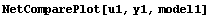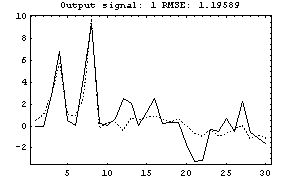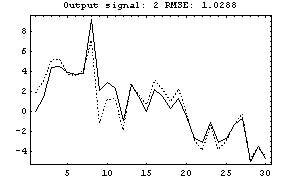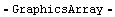Q: How can I implement neural networks in other programming languages?

You can convert a neural network to program code in either C or Fortran using the Mathematica commands CForm or FortranForm. This code can then be inserted into external programs. Here is an illustration of how to do this conversion on a feedforward network.Generate some data.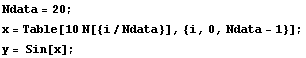Generate a network.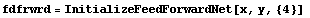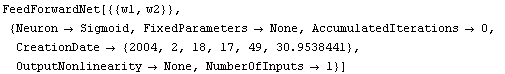You can now obtain the program code by first producing a symbolic expression of the network and then using the Mathematica commands CForm or FortranForm.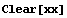The input to the network should be a list of symbols, one symbol for each input of the neural network. In this example there is only one input.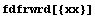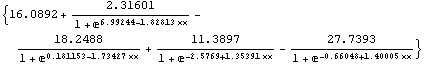You can then produce the C code using the CForm command.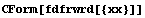List(16.089224963527823 + 2.316011672835048/
(1 + Power(E,6.99243912182352 - 1.8281339324526809*xx)) -
18.248785203200498/(1 + Power(E,0.18115270188825505 - 1.7342669596549924*xx)) +
11.389669725578603/(1 + Power(E,-2.5768975618284284 + 1.353905670349015*xx)) -
27.739273072885055/(1 + Power(E,-0.6604795224465034 + 1.4000466309075252*xx)))

Similarly, to create Fortran code use the FortranForm command.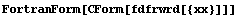CForm(List(16.089224963527823 +
-    2.316011672835048/(1 + E**(6.99243912182352 - 1.8281339324526809*xx)) -
-    18.248785203200498/(1 + E**(0.18115270188825505 - 1.7342669596549924*xx)) +
-    11.389669725578603/(1 + E**(-2.5768975618284284 + 1.353905670349015*xx)) -
-    27.739273072885055/(1 + E**(-0.6604795224465034 + 1.4000466309075252*xx))))